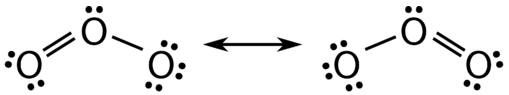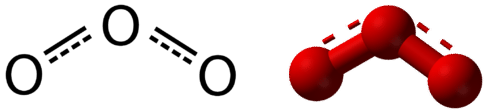# 3.6: Resonance

$$\newcommand{\vecs}{\overset { \rightharpoonup} {\mathbf{#1}} }$$ $$\newcommand{\vecd}{\overset{-\!-\!\rightharpoonup}{\vphantom{a}\smash {#1}}}$$$$\newcommand{\id}{\mathrm{id}}$$ $$\newcommand{\Span}{\mathrm{span}}$$ $$\newcommand{\kernel}{\mathrm{null}\,}$$ $$\newcommand{\range}{\mathrm{range}\,}$$ $$\newcommand{\RealPart}{\mathrm{Re}}$$ $$\newcommand{\ImaginaryPart}{\mathrm{Im}}$$ $$\newcommand{\Argument}{\mathrm{Arg}}$$ $$\newcommand{\norm}{\| #1 \|}$$ $$\newcommand{\inner}{\langle #1, #2 \rangle}$$ $$\newcommand{\Span}{\mathrm{span}}$$ $$\newcommand{\id}{\mathrm{id}}$$ $$\newcommand{\Span}{\mathrm{span}}$$ $$\newcommand{\kernel}{\mathrm{null}\,}$$ $$\newcommand{\range}{\mathrm{range}\,}$$ $$\newcommand{\RealPart}{\mathrm{Re}}$$ $$\newcommand{\ImaginaryPart}{\mathrm{Im}}$$ $$\newcommand{\Argument}{\mathrm{Arg}}$$ $$\newcommand{\norm}{\| #1 \|}$$ $$\newcommand{\inner}{\langle #1, #2 \rangle}$$ $$\newcommand{\Span}{\mathrm{span}}$$$$\newcommand{\AA}{\unicode[.8,0]{x212B}}$$

Learning Objectives
• Define resonance
• Identify whether a molecule has resonance

## Resonance

There are some cases in which more than one viable Lewis structure can be drawn for a molecule. An example is the ozone, $$\left( \ce{O_3} \right)$$. Ozone a gas present in different regions of the atmosphere. When ozone is present in the troposphere it is an air pollutant that is harmful to living species and a greenhouse gas that contributes to climate change.  Ozone in the stratosphere helps protects the Earth from overexposure to UV radiation. There are a total of 18 valence electrons in the structure, 6 valence electrons from each oxygen atom, so the following two structures are possible in Figure $$\PageIndex{1}$$.Figure $$\PageIndex{1}$$: Resonance forms of ozone. Note the use of the double-headed arrow.

The structure on the left ($$\PageIndex{1}$$) can be converted to the structure on the right by a shifting of electrons without altering the positions of the atoms.

It was once thought that the structure of a molecule such as $$\ce{O_3}$$ consisted of one single bond and one double bond which then shifted back and forth as shown above. However, further studies showed that the two bonds are identical. Any double covalent bond between two given atoms is typically shorter than a single covalent bond. Studies of the $$\ce{O_3}$$ and other similar molecules showed that the bonds were identical in length. Interestingly, the length of the bond is in between the lengths expected for an $$\ce{O-O}$$ single bond and a double bond.

Resonance is the use of two or more valid (or contributing) Lewis structures to represent the covalent bonding in a molecule. The valid structures are referred to as resonance structures. It is now understood that the actual structure of a molecule which displays resonance is that of an average or a hybrid of all the valid resonance structures. In the case of the $$\ce{O_3}$$ molecule, each of the covalent bonds between $$\ce{O}$$ atoms is best thought of as being "one and a half" bonds, as opposed to either a pure single bond or a pure double bond. This "half-bond" can be shown as a dotted line in both the Lewis structure and the molecular model (Figure $$\PageIndex{2}$$).Figure $$\PageIndex{2}$$: "Half-bond" model of ozone molecule. This is a better description of the electornic structure of ozone than either of the resonance structures in  Figure $$\PageIndex{1}$$.

## Summary

•  It is possible to draw resonance structures for molecules that are not viable structures. Additional guidelines for assessing the validity of a Lewis structure beyond the octet rule (or duet for hydrogen) are beyond the scope of this course.

• The resonance structures only differ in the placement of electrons – the connectivity of the atoms in the molecule remains the same between resonance structures.